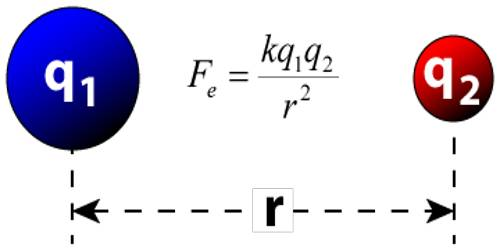# Limitation of Coulomb’s Law

Limitation of Coulomb’s Law

Coulomb’s law is an extended method of the quantity 4π in MKS unit. This method is proposed by Oliver Heaviside and Lorentz in 1995.

Apparently, it appears that inclusion of the quantity 4π in Coulomb’s law creates some complication, although that quantity can be included deliberately into the equation. In other extensively used equation where the presence of the quantity 4π in absence of spherical symmetry and presence of the quantity 2π in absence of cylindrical symmetry, creates complication. In order to simplify those equations, an appropriate method of the unit is needed. For example in CGS unit, the capacitance is, C = A / 4πD e.s.u.

But in MKS or SI unit C =4πε0A / 4πd = (ε0A / d) Farad

From the idea of induction, it is seen that, the number at tubes of force passing through the area located perpendicular to the direction of electric field = ε E, where E = Electric field, ε = permittivity of the medium. The total induction in a plane can be calculated by Gauss’s law in that plane but total induction cannot be calculated by Coulomb’s law. Again, other than point charge magnitude of applied force in any other charged bodies cannot be determined by Coulomb’s law.

Coulomb’s law is valid if the average number of solvent molecules between the two interesting charge particles should be large.

In short,

Coulomb’s Law is derived under definite assumptions and can’t be used liberally like other general formulas. The law is limited to the following points:

• Coulomb’s law is valid if the average number of solvent molecules between the two interesting charge particles should be large.
• Coulomb’s law is valid if the point charges are at rest.
• Coulomb’s Law is applied to the point. If charged bodies are of limited dimension such that they can’t be regarded as a point charge then Coulomb’s Law is not valid. So, Coulomb’s law is not valid for distance less than 10-15
• Coulomb’s law obeys inverse square law. It’s only appropriate in dose cases where inverse square law is obeyed.
• It is only applicable for point charges at rest. We can use the formula if the charges are static (in rest position). If a charge is in motion Coulomb’s law of electrostatics.
• It is only valid when the solvent molecules between particles are suitably bigger than both the charges.
• The formula is easy to use while dealing with charges of regular and smooth shape, and it becomes too complex to deal with charges having irregular shapes.
• It is difficult to apply Coulomb’s law when the charges are in an arbitrary shape. Hence, we cannot determine the value of distance ‘d’ between the charges when they are in an arbitrary shape. Here, the distance, r cannot be resolute correctly as the centers of arbitrarily shaped charged bodies cannot be recognized exactly.